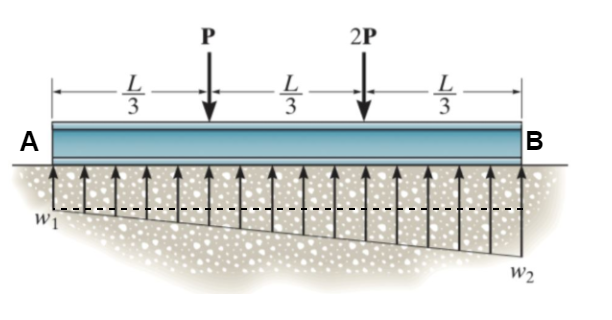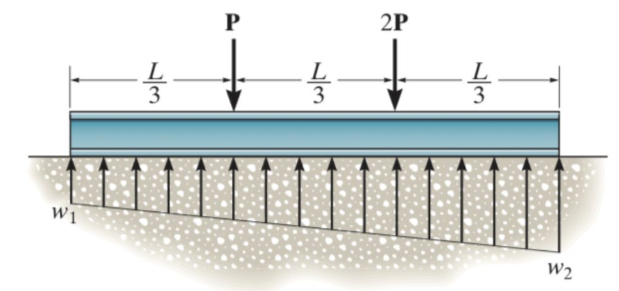# Problem: If P = 500 lb and L = 12 ft., what are the magnitudes of w1, w2 ?

###### FREE Expert Solution

Relabel the figure as:From the equilibrium condition:

$\overline{){\mathbf{\Sigma }}{\mathbf{F}}{\mathbf{=}}{\mathbf{0}}}$

The vertical equilibrium:

The varying load given is divided into a rectangle and a triangular load.

We have:

w1L + (1/2)(w2 - w1)L = P + 2P

w1L + (1/2)w2L - (1/2)w1L = 3P

(1/2)w1 + (1/2)w2L = 3P/L

w1 + w2 = 6P/L

Considering the moment at B:

w1L + (1/2)L + (1/2)(w2 - w1)L + (1/3)L = P(2/3)L + 2P(1/3)L

w1L2/2 + w2L2/6 - w1L2/6 = 4PL/3

2w1 + w2 = 8P/L

93% (356 ratings)###### Problem DetailsIf P = 500 lb and L = 12 ft., what are the magnitudes of w1, w2 ?

Frequently Asked Questions

What scientific concept do you need to know in order to solve this problem?

Our tutors have indicated that to solve this problem you will need to apply the More 2D Equilibrium Problems concept. You can view video lessons to learn More 2D Equilibrium Problems. Or if you need more More 2D Equilibrium Problems practice, you can also practice More 2D Equilibrium Problems practice problems.